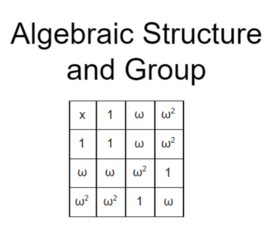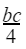# Algebraic Structure and GroupAlgebraic Structure

A set together with a binary operation gives rise to what is known as an algebraic structure.

Given a, b, c as the elements of the set G, the algebraic structure (G,*) maybe:

1. Closed

2. Commutative

3. Associative

4. Existing of Identity element

5. Existing of inverse element

Group

The non- empty set G together with the binary operation ౦ is said to be a group if it satisfies the following axioms/ properties:

1. Closure Axiom:

For elements a, b ∈ G, a ౦ b ∈ G

1. Associative Axiom:

For a, b, c ∈ G, (a ౦ b) ౦ c = a ౦ (b ౦ c)

1. Existence of Identity Element:

For a ∈ G, there exists an identity element e ∈ G, such that, a ౦ e = e ౦ a = a

1. Existence of Inverse Element:

For each element a ∈ G, there exists an inverse element a-1 ∈ G, such that, a ౦ a-1 = a-1 ౦ a = e

Exercise:

Prove that G= {1, ω, ω2}, where ω is a cube root of unity, is a group under multiplication.

Solution:

The cayley’s table is as follows:

Note that: ω3=1

 х 1 ω ω2 1 1 ω ω2 ω ω ω2 1 ω2 ω2 1 ω

i) Closure Axiom  It is clear from the table above that all the elements of the table belong to G. So, the closure axiom is satisfied.

ii) Associative Axiom

∵ 1, ω, ω2 ∈ G

∴ (1 х ω) х ω2 = 1 х (ω х ω2

or,   ω х ω2 = 1 х ω3

or,   1=1 (True)

Similarly, the axiom is true for other elements too. So, associativity is satisfied.

iii) Existence of Identity element

Here, 1 is the identity element  as 1 fixes all other elements and also 1 ∈ G. So, the existence of identity is satisfied.

iv) Existence of inverse element

Here, 1 х 1 = 1. So, 1 is the inverse of 1

ω х ω2 = ω2 х ω = 1. So, ω is the inverse of ω2 and vice versa.

All three elements lie within G.

So, the existence of an inverse element is also satisfied.

Hence, all the properties of a group are satisfied. So, G is a group.

Finite and Infinite Group

A group is finite if it has a finite number of elements and a group is called infinite if it has infinite number of elements.

The set S= {1, -1} is a finite group under multiplication and its order is 2.

The set of integers is an infinite group under addition.

Trivial Group

A group consisting of only one element is a trivial group.

The set G= {0} ωith addition is a trivial group.

Abelian Group

The group G is said to be an abelian group if it satisfies commutative axiom,

i.e. for all a, b ∈ G, a ౦ b = b ౦ a

Exercise 2: Show that the set of all (+)ve rational numbers form an abelian group under composition defined by a ౦ b =Solution:

Let Q+ = set of (+)ve rational numbers

Q+ = {1, 2, 3, ……,}

1) Closure Property:

For a, b ∈ Q+

a ౦ b =∈ Q+ as the product and division of (+)ve rational numbers is always a (+)ve rational number.

So, the closure property is satisfied.

2) Associative Property

Let a, b, c ∈ Q+

Then, (a ౦ b) ౦ c = a ౦ (b ౦ c)౦ c = a ౦∴abc= abc (True)

So, the associative property is also satisfied.

3) Commutative property

Let a, b ∈ Q+

a ౦ b = b ౦ a=∴ab= ab (True)

So, the commutative property is also satisfied.

3) Existence of Identity Element

Let e be the identity element

Then, a ౦ e = a

or,= a

or, e = 4 ∈ Q+

And this identity element also lies in Q+.

So, the existence of the identity element is satisfied.

4) Existence of Inverse Element

Let a ∈ Q+ and a-1 be the inverse of a

Then, a ౦ a-1 = e

or,= 4

or, a-1∈ Q+

So, the existence of an inverse element is also satisfied.

Hence, Q+ is an abelian group.